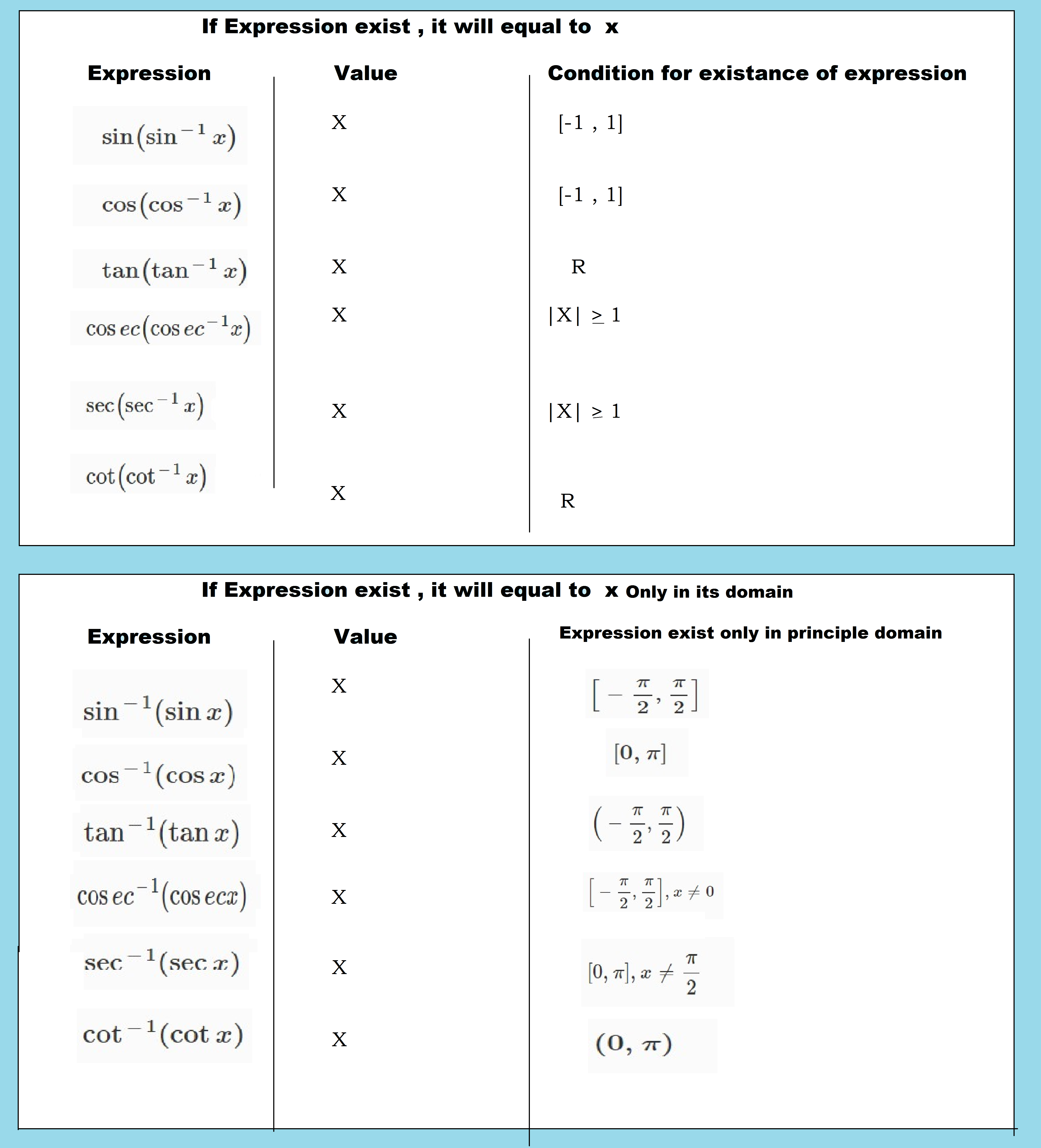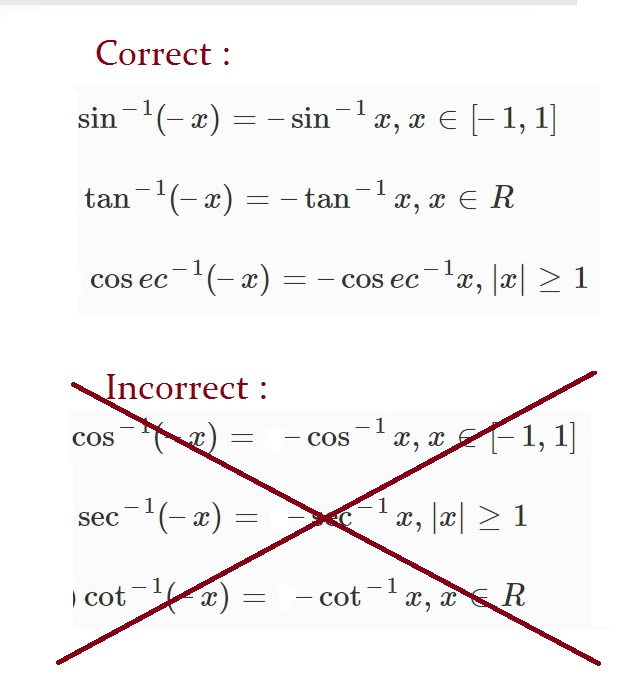Mathematics Properties of Inverse Trigonometric Functions For CBSE-NCERT
Click for Only Video

### Topic covered

star Properties of inverse trigonometric function

### Property 1 :\color{red} \ox \color{red} (COMMON \ CONFUSION)

sin (sin^-1 x) = x, x ∈ [– 1, 1] and sin^-1 (sin x) = x, x ∈ [ - pi/2, pi/2]

cos (cos^-1 x) = x, x ∈ [– 1, 1] and cos ^-1 (cos x) = x, x ∈ [ 0,pi]

tan (tan^-1 x) = x, x ∈ R and tan^-1 (tan x) = x, x ∈ ( - pi/2, pi/2)

cosec (cosec^-1 x) = x, |x| ge 1 and cosec^-1 (cosec x) = x, x ∈ [ - pi/2, pi/2] , xne0

sec (sec^-1 x) = x, |x| ge 1 and sec^-1 (sec x) = x, x ∈ [ 0, pi], x ne pi/2

cot (cot^-1 x) = x, x ∈ R and cot^-1 (cot x) = x, x ∈ ( 0,pi)

\color{purple}ul(✓✓) \color{purple} "ALERT"

sin(sin^(-1)1) = 1
sin(sin^(-1)2) ne 2 (Firstly given question is wrong as 2 isn't in domain)

\color { maroon} ® \color{maroon} ul (" REMEMBER") : So if sin(sin^(-1)x) is given means x always will be in domain,So we can write directly equal to x

Property 1 :

• sin ^-1 \ \1/x = cosec^-1 x , x >= 1  or  x <= -1

• cos^-1 \ \ 1/x = sec^-1 x, x ≥ 1 or x ≤ – 1

• tan ^-1 \ \ 1/x = cot^-1 x , x > 0

To prove the first result, we put cosec^-1 x = y, i.e., x = cosec y

Therefore 1/x = sin y

Hence sin^-1 \ \1/x = y

or  sin ^-1 \ \1/x = cosec^-1 x

Similarly, we can prove the other parts.

### Property 2 :

(i) sin^-1 (–x) = – sin^-1 x, x ∈ [– 1, 1]

(ii) tan^-1 (–x) = – tan^-1 x, x ∈ R

(iii) cosec^-1 (–x) = – cosec^-1 x, | x | ≥ 1

Let sin^-1 (–x) = y, i.e., –x = sin y so that x = – sin y, i.e., x = sin (–y).

Hence sin^-1 x = – y = – sin^-1 (–x)

Therefore sin^-1 (–x) = – sin^-1 x

Similarly, we can prove the other parts.

### Property 3 :(i) cos^-1 (–x) = π – cos^-1 x, x ∈ [– 1, 1]

(ii) sec^-1 (–x) = π – sec^-1 x, | x | ≥ 1

(iii) cot^-1 (–x) = π – cot^-1 x, x ∈ R

Let cos^-1 (–x) = y i.e., – x = cos y so that x = – cos y = cos (π – y)

Therefore cos^-1 x = π – y = π – cos^-1 (–x)

Hence cos^-1 (–x) = π – cos^-1 x

Similarly, we can prove the other parts.

### Property 4 :

(i) sin^-1 x + cos^-1 x = pi/2, x ∈ [– 1, 1]

(ii) tan^-1 x + cot^-1 x = π/2, x ∈ R

(iii) cosec^-1 x + sec^-1 x = pi/2 , | x| >= 1

Let sin^-1 x = y. Then x = sin y = cos ( pi/2 - y)

Therefore cos^-1 x = pi/2 - y = pi/2 - sin ^-1 x

Hence sin^-1 x + cos^-1 x = pi/2

Similarly, we can prove the other parts.

### Property 5

(i) tan^-1x + tan^-1 y = tan^-1 \ \( x +y)/( 1 - xy) , xy < 1

(ii) tan^-1x – tan^-1 y = tan^-1 \ \( x - y)/( 1 + xy), xy > -1

(iii) 2tan^-1x = tan^-1\ \ (2x ) /( 1- x^2) , | x | < 1

Let tan^-1 x = θ and tan^-1 y = phi. Then x = tan θ, y = tan phi

Now tan ( theta + pi) = ( tan theta + tan phi )/( 1- tan theta tan phi ) = ( x + y) /( 1- xy)

This gives  theta + phi = tan ^-1 \ \ ( x+y) /(1 -xy)

Hence  tan ^-1 a + tan ^-1 y = tan^-1 \ \ ( x +y) /(1 -xy)

In the above result, if we replace y by – y, we get the second result and by replacing y by x, we get the third result.

### Property 6 :

(i) 2tan^-1 x = sin^-1 \ \( 2x) /( 1 + x^2) , | x| <=1

(ii) 2tan^-1 x = cos^-1 \ \( 1- x^2 ) /( 1 +x^2 ) , x >= 0

(iii) 2 tan^-1 x = tan^-1 \ \ (2 x) /(1 -x^2 ) . -1 < x < 1

Let tan^-1 x = y, then x = tan y. Now

sin^-1 \ \ ( 2 x) /( 1 + x^2) = sin^-1 \ \ ( 2 tan y) /( 1 + tan^2 y)

= sin^-1 (sin 2y) = 2y = 2tan^-1 x

Also cos^-1 \ \ ( 1 - x^2 ) /( 1 +x^2) = cos^-1 \ \ ( 1 - tan^2y)/( 1 + tan^2y) = cos ^-1 \ \ ( cos 2y ) = 2y = 2 tan ^-1 x

\color{green} ✍️ \color{green} \mathbf(KEY \ CONCEPT) : If we ignore the domain then

2tan^-1 x = sin^-1 \ \( 2x) /( 1 + x^2) = cos^-1 \ \( 1- x^2 ) /( 1 +x^2 ) = tan^-1 \ \ (2 x) /(1 -x^2 )

(iii) Can be worked out similarly.
Q 31655234653 sin^(-1) ( (2x)/( 1+x^2) ) -4 cos^(-1) ( (1-x^2 )/(1+x^2) ) + 2 tan^(-1) ( (2x)/( 1-x^2) ) = pi/3 .Solution:

We have

 3 sin^(-1) ( (2x)/( 1+x^2) ) -4 cos^(-1) ( (1-x^2 )/(1+x^2) ) +2 tan^(-1) ( (2x)/( 1-x^2) ) = pi/3

Put x = tan theta in (1) , then

3 sin^(-1) ( (2 tan theta )/( 1+ tan^2 theta) ) -4 cos^(-1) ( ( 1- tan^2 theta)/ (1+ tan^2 theta)) + 2 tan^(-1) ( (2 tan theta)/ ( 1- tan^2 theta) ) = pi/3

=> 3 sin^(-1) (sin 2 theta) -4 cos^(-1) (cos 2 theta) + 2 tan^(-1 ) (tan 2 theta) = p i/3

=> 3 (2 theta) -4 (2 theta) + 2 (2 theta) = pi/3

=> 10 theta - 8 theta = pi/3

=> 2 theta = pi/3

=> theta = pi/6

=> tan^(-1) x = pi/6

=> x= tan pi/6

=> x= 1/(sqrt 3)

### NCERT Examples

Q 3163345245Show that

sin^(-1) (2x sqrt(1-x^2))= 2 sin^(-1) x , - 1/(sqrt 2) le x le 1/ sqrt(2)Solution:

Let x = sin θ. Then sin^(–1) x = θ. We have

sin^(-1) (2x sqrt(1-x^2)) = sin^(-1) ( 2 sin theta sqrt(1-sin^2 theta))

= sin^(–1) (2sinθ cosθ) = sin^(–1) (sin2θ) = 2θ

= 2 sin^(–1) x
Q 3143445343Show that

sin^(-1) (2x sqrt(1-x^2))= 2 cos^(-1) x , - 1/(sqrt 2) le x le 1Solution:

Take x = cos θ, then proceeding as above,

sin^(–1) (2x sqrt(1− x^2 )) = sin^(–1) (2costheta . sintheta)
= sin^(–1)sin 2theta =2 theta = 2cos^(-1)x

we get, sin^(–1) (2x sqrt(1− x^2 ))= 2 cos^(–1) x
Q 3163045845Show that tan^(–1) \ 1/2 + tan^(-1) \ 2/11 = tan^(-1) \ 3/4Solution:

By property (i) , we have

L.H.S. = tan^(–1) \ 1/2 + tan^(-1) \ 2/11 = tan^(-1) \ (1/2 + 2/11)/(1- 1/2 xx 2/11)= tan^(-1) \ 15/20 = tan^(-1) \ 3/4 = R.H.S
Q 3113145940Write cot^(-1) (1/(sqrt(x^2-1))) , |x| > 1 in the simplest form.Solution:

Let x = sec θ, then sqrt(x^2-1)= sqrt(sec^2 theta-1)= tan theta

Therefore cot^(-1) \ 1/ (sqrt (x^2-1)) = cot^(-1) (cot theta)= theta = sec^(-1) x, which is the simplest form.
Q 3103145948Express tan^(-1) ((cos x)/(1-sin x)), - pi/2 < x < pi/2 in the simplest form.Solution:

We write tan^(-1) ((cos x)/(1- sin x)) = tan^(-1) [ (cos^2 \ x/2 - sin^2 \ x/2)/(cos^2 \ x/2 + sin^2 \ x/2 cos \ x/2)]

= tan^(-1) [ ((cos \ x/2 + sin \ x/2 )(cos \ x/2 - sin \ x/2))/(cos \ x/2 - sin \ x/2 )^2)]

=tan^(-1)[ (cos \ x/2 + sin \ x/2)/(cos \ x/2 - sin \ x/2)]= tan ^(-1) [ (1+ tan \ x/2)/(1- tan \ x/2)]

= tan ^(-1) [ tan (pi/4 + x/2)] = pi/4 + x/2
Q 3133145942Prove that tan^(–1) x+ tan^(-1) \ (2x)/(1-x^2)= tan^(-1) \ ((3x-x^3)/(1-3x^2)), |x| < 1/(sqrt 3)Solution:

Let x = tan θ. Then θ = tan^(–1) x. We have

R.H.S = tan^(-1) ((3x-x^3)/(1-3x^2)) = tan^(-1) ((3 tan theta - tan^3 theta)/(1- 3 tan^2 theta))

= tan^(–1) (tan3θ) = 3θ = 3tan^(–1) x = tan^(–1) x + 2 tan^(–1) x

= tan^(–1) x + tan^(–1) \ (2x)/(1-x^2)= L.H.S.
Q 3153145944Find the value of cos (sec^(–1) x + cosec^(–1) x), | x | ≥ 1Solution:

We have cos (sec^(–1) x + cosec^(–1) x) = cos (pi/2)=0